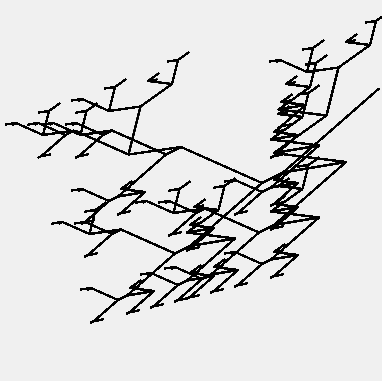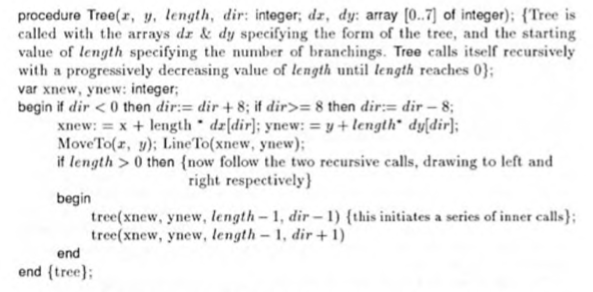# Recursive problem

I have a recursive method:

``````Public Sub Tree(x as Integer, y as Integer, length as Integer, dir as Integer, Optional DX() as Integer, Optional DY() as Integer)
'initialize the graphic class

Var G As Graphics
Var Xnew, Ynew As Integer

'check dir
If dir < 0 Then dir = dir + 8
If dir >= 8 Then Dir = Dir -8

'calculate new position
Xnew = X + Length * DX(Dir)
Ynew = Y + length * DY(Dir)

'move to start point
Morph.MoveToPoint(x,y)

'draw a line to new position

'recursive calls for new branches
If length > 0 Then
Tree(Xnew,Ynew, length-1, dir-1)
Tree(Xnew,Ynew, length-1, dir+1)
End If

'draw the path
G.DrawPath(Morph)

End Sub

``````

The parameters DX() and DY() are filled with integers. Initial run of the Tree() method is ok, but as soon as the recursive call is made these are both NIL. Parameters are declared as properties in the Window. How come?

count the parameters if you call tree again …

It is probably because then you call Tree into Tree you don’t pass DX DY as parameters.

Ehhh■■■■…

I got it working now but I face another problem:

``````Public Sub Tree(G as Graphics, x as Integer, y as Integer, length as Integer, dir as Integer, Optional DX() as Integer, Optional DY() as Integer)
'initialize the graphic class

Var Xnew, Ynew As Integer

'check dir
If dir < 0 Then dir = dir + 8
If dir >= 8 Then Dir = Dir -8

'calculate new position
Xnew = X + Length * DX(Dir)
Ynew = Y + length * DY(Dir)

'move to start point
Morph.MoveToPoint(x,y)

'draw a line to new position

'recursive calls for new branches
If length > 0 Then
Tree(G, Xnew,Ynew, length-1, dir-1,DX,DY)
Tree(G, Xnew,Ynew, length-1, dir+1,DX,DY)
End If

'draw the path
G.DrawPath(Morph)

End Sub

``````

The second recursive call is never reached. But I want both calls to be executed as long as Length > 0. How to do that?

What are the initial values?
What do you expect to draw with them?

It is an implementation of a ‘biomorph’, originally developed by Richard Dawkins in Pascal.

It draws a more or less biological figure, controlled by ‘genes’ in the DX and DY arrays (values from -9 to 9). The first recursive call draws the left side and the second (where ‘dir+1’) draws the right side, which is identical but mirrored.

But because Length reaches zero by the first recursive calls, the second part is never reached.
In Pascal they are enclosed by a begin and end statement so they get called both (I guess)The recursive call won’t be modifying length. So each recursive call to Tree should be executed.

Changed the code,

``````'initialize the graphic class

Var Xnew, Ynew As Integer

Counter = Counter + 1
'check dir
If dir < 0 Then dir = dir + 8
If dir >= 8 Then Dir = Dir -8

'calculate new position
Xnew = X + Length * DX(Dir)
Ynew = Y + length * DY(Dir)

'move to start point
Morph.MoveToPoint(x,y)

'draw a line to new position
Length=Length-1
'recursive calls for new branches
If length > 0 Then
Tree(G, Xnew,Ynew, length, dir-1,DX,DY)
Tree(G, Xnew,Ynew, length, dir+1,DX,DY)
End If

'draw the path
G.DrawPath(Morph)
``````

but still the second call isn’t reached…

Length in Tree is not modified by the recursive call.

Now, when you say that the second call to Tree is not reached, what do you mean? What gets drawn? Does the program loop for ever? Does it crash?

Ok. It finishes nicely, but only the first recursive call is executed and again, and again, until Length = 0. I see that in the debugger. I start with 6, 5 4 3 2 1 and with zero the If statement is not executed anymore so G.DrawPath gets called, drawing the lines.

Below the original codeSo what happens if you remove the second recursive call (or comment it out)?

Hey, that is interesting. I get a picture that has half of the complexity.

So, why did you think the second call was not being made?

1 Like

Because I followed the execution in the debugger. I never saw it getting highlighted. Thanks for that. But still it draws only half of the picture…

Don’t forget that when Tree returns from the length=0 value, it goes up one level and then calls the other Tree. That should run and then return. You may need to step several times to get right back up the recursive calls and perhaps the debugger doesn’t show that very well.

Very interesting, thanks!

Looks like a variation of Lindenmeyer- (or L-) system which I can make in JWildfire:

Looks like it, but Dawkin’s algorithm should produce this kind of critters:

My code produces only the left half1 Like

Ah yes I remember running that code more than 30 years ago.